Mathematics

# Formulas of Coordinate Geometry

298 views

 1 Introduction 2 Names related to coordinate geometry 3 Distance formula 4 Section formula 5 Midpoint theorem 6 Lines 7 Some applications in real life 8 Summary 9 FAQs

22 December 2020

## Introduction

Coordinate geometry is also known as analytic geometry. Cartesian geometry also stands for coordinate geometry.

It is a branch of geometry. It expresses the position of points on a plane using numbers having ordered pairs. Thus, coordinate geometry is the study of geometry based on coordinate points. We find how geometry, algebra, graphs, curves, and lines are related in expressions to solve problems.

Coordinate geometry is used to find:

(i) The distance between two points.

(ii) The midpoint of a line.

(iii) Sections of a line in a specified ratio.

(iv) The area of the triangle in a plane.

(v) The angle between the two lines.

These problems are two-dimensional.

## Names related to coordinate geometry

### 1) Coordinate plane

It is a two-dimensional plane formed by two lines, which are at 90⁰ to each other. Of these, the horizontal lines are X-axis, and the vertical line is Y-axis. The intersection of these is the origin.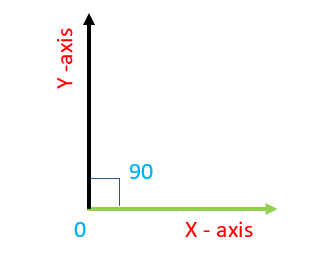### 2) Coordinates

Coordinates are a set of two values showing the correct location of a point in the plane. In the figure shown, P(x,y) is a point on the plane X-Y.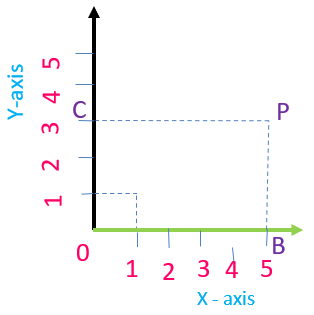The distance CP = 5 is the distance of the point from the Y-axis. It is X-coordinate or abscissa. The distance is the distance of point P from the X-axis and is called Y coordinate or ordinate.

## Distance formula

The distance formula is used to calculate the distance between two points on the plane. To find the distance between two points P(x,y) and Q(x,y) shown in the figure, we use the Pythagoras theorem,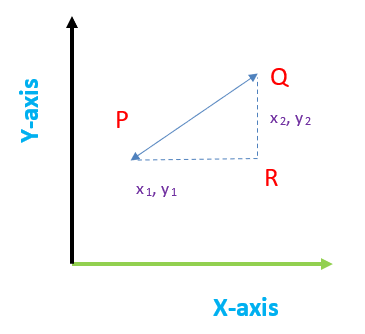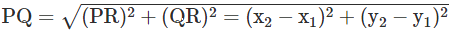Thus, we calculate the distance. This is a two-point formula theory.

 Example:

Find the distance between 2 points A(-4,3) and B(6,-4)

Solution:

Point A : x1 =−4, y1=3

Point B: x2 =6, y2=−4

Distance formula $$=$$ distance between two points A and B   $$=\sqrt{ (x_2-x_1)^2 + (y_2-y_1)^2}$$## Section formula

Section formula is used to arrive at each portion of aline’s lengths when the line is divided in a specified ratio say in $$\text{p : q}$$ ratio.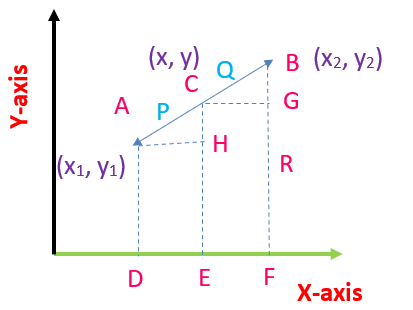Proof:

In the figure to divide the line AB internally in the ratio $$\text{p : q}$$

Draw vertical lines at D, E, and F to points A $$\rm{}(x_1, y_1), C (x,y),$$ and B $$\rm{}(x_2, y_2).$$ Also draw horizontal lines parallel to X-axis from points A, C, and B to meet the lines at the points H and G.

Triangles AHC and CGB are similar.

Hence, $$\frac{AC}{CB}=\frac{AH}{CG}=\frac{CH}{BG}$$

$$\frac{p}{q}=\frac{x-x_1}{x_2-x}$$ and $$\frac{x-y_1}{y_2-y}$$

$$\text{i.e., }p (x_2-x) = q (x-x_1) \text{ and } p (y_2-y) = q (y-y_1) \\\\\qquad \quad\;\;\;\;= px_2-px = qx-qx_1$$

$$\text{i.e., }px +qx = px_2 + qx_1\\x(p+q) = px_2 +qx_1$$

\begin{align}x=\frac{p x_{2}+q x_{1}}{p+q}\end{align}

similarly, \begin{align}y=\frac{p y_{2}+q y_{1}}{p+q}\end{align} can be proved.

Thus, point C is \begin{align}\text{C }\left(\frac{p x_{2}+q x_{1}}{p+q}, \frac{p y_{2}+q y_{1}}{p+q}\right)\end{align}

(i) When C is midpoint of line AB, then $$\text{AC = CB. i.e., p = q =1}$$

Then the midpoint is \begin{align}\text{C }\left(\frac{x_{2}+x_{1}}{2}, \frac{y_{2}+y_{1}}{2}\right)\end{align}

## Midpoint theorem

When a line is internally divided into two equal sections, the dividing point is the line’s midpoint.

Let AB be the line with $$\rm{}A (x_1, y_1)$$and $$\rm{}B (x_2, y_2)$$

Then the midpoint of the line $$\rm{AB} = \left(\frac{x_2+x_1}{2}, \frac{y_2+y1}{2}\right)$$

(ii) When the line is divided in p:q ratio externally,

the point is $$\left(\frac{px_2+qx_1}{p-q}, \frac{py_2+qy_1}{p-y}\right)$$

 Example:

Mark the 2 points $$\rm{}A (10, 2)$$ and $$\rm{}B (7,1)$$ in a sketch. What are the X coordinates and Y coordinates for the points?

i) Find the length of the line AB.

ii) Find the midpoint of the line AB

iii) Find the point C, which divides the line into parts in the ratio $$2:1$$

Solution:

X coordinates 10 for point A

7 for point B

Y coordinates 2 for point A

1 for point B

i) Length of the line $$= \sqrt{(10-7)^2 + (2-1)^2} = \sqrt{9+1} =\sqrt{10}$$ units.

ii) Midpoint of the line \begin{align}=\frac{x_2+x_1}{2},\frac{y_2+y_1}{2}=\frac{10+7}{2},\frac{2+1}{2}=8.5, 1.5. \end{align} .

iii) Division of the line in $$2:1$$ ratio internally.

Dividing point by section formula \begin{align}=\frac{px_2+qx_1}{p+q}, \frac{py_2+qy_1}{p+q}\end{align}

Where $$p = 2, q = 1$$

\begin{align}x_2 &= 7, \;x_1 = 10\\\\y_1 &= 2, \;y_2= 1\end{align}

\begin{align}\text{the dividing point }&=\frac{2 * 7+1 * 10}{2+1}, \frac{2 * 1+1 * 2}{2+1} \\&=\frac{24}{3}, \frac{4}{3}\\&=8,1\left(\frac{1}{3}\right) .\end{align}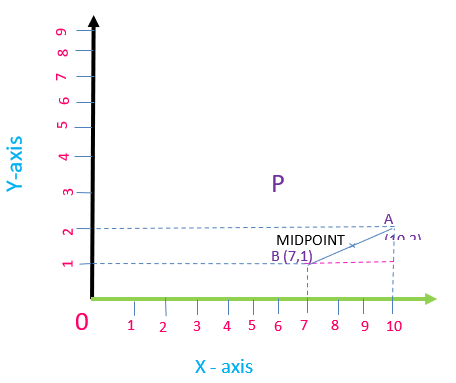Graphs are plotted on a plane for equations. The number line is called the cartesian plane, which has four quadrants formed by two perpendicular lines. The vertical line is Y-axis, and the horizontal line is X-axis.

The cartesian plane is as shown in the figure. A point P (x, y) is marked. It has $$x = 5,$$ which is

X - coordinate or abscissa, and $$y = 3,$$ which is Y- coordinate or ordinate.

The coordinate or cartesian plane has four quadrants as shown above.

Equation of a line in cartesian plane

(i) General form $$\rm{}= px + qy + r = 0$$

(ii) Slope intercept form $$\rm{}= y = mx + c$$

where $$\rm{m} =$$ slope of the line and $$\rm{}c = y$$- intercept

$$x, y =$$ values of the point.

(iii) Slope of line: $$\rm{}y = mx + c$$ is ‘$$\rm{m}$$

## Lines

### The Slope of a line

Let px + qy + r = 0be the given line.

General form is $$\rm{}qy = - px - r$$

$$y = - \frac{p}{q}x-\frac{r}{q}.$$

Here slope ‘$$\rm{m}$$’ is $$– \frac{p}{q}.$$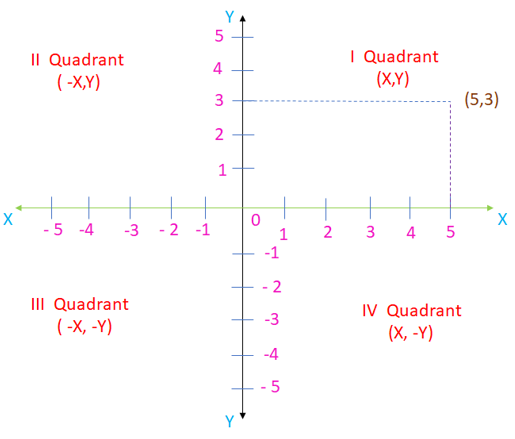### Cases of two lines

i) The Angle between two lines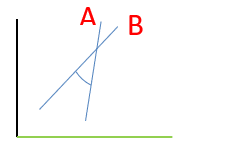Let $$\rm{}m_1 =$$slope of the line A and $$\rm{}m_2 =$$ slope of the line B

$$θ =$$ angle at the intersection of the lines

Then $$\text{tan θ }= \rm{}m_1 – m_2 (1 + m_1m_2)$$

ii) Parallel lines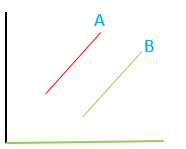Angle between the lines is zero.

$$\rm{}m_1 = m_2 = m$$ (slopes are equal)

\begin{align}\text{tan } θ &=\rm{} m_1 – m_2 + m_1m_2\\&= \rm{}m - m_1 + m_2 = 0\\ θ&= 0 \end{align}

 Example:

Find the equation of a line parallel to the line $$\rm{}4x +5y = 7$$ and passing through a point $$(2,3)$$.

Solution:

Given line, $$\rm{}4x + 5y = 7$$

$$\rm{}i.e., 4x + 5y = k$$

at point $$(2, 3), 4 \times 2 + 5 \times 3 = 23,$$

the equation of the line is $$\rm{}4x + 5y = 23.$$

iii) Lines perpendicular to each

$$\rm{}m_1.m_2 = -1$$

$$\rm{}tan = m_1 - m_2 (1 + (-1)) = m_1 - m_2 (0),$$ undefined

$$θ = 90^\circ$$.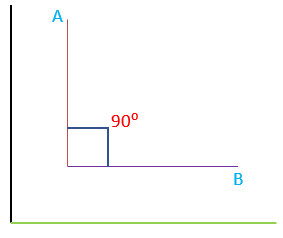vi) Area of triangle

$$\frac{1}{2}(x_1(y_2 – y_3) + x_2(y_3 – y_1) + x_3(y_1 – y_2))$$

Where $$(x_1, y_1), (x_2, y_2),$$ and $$(x_3, y_3)$$ are vertices of the triangle.

The vertices are co-linear if the area of the triangle is zero.

## Some applications of coordinate geometry in real - life conditions

(i) In digital devices to position the cursor.

(ii) To know the location of planes (in aviation)

(iii) GPS – in navigation and maps

(iv) Locations may be mapped geographically concerning the longitudes and latitudes.

## Summary

Coordinate geometry is the branch of mathematics, comprising dimensional quantities, coordinates, coordinate plane. It rules algebraic expressions, mathematical problems with graphs.

In this blog we discuss about:

• Names related to coordinate geometry
• Distance Formula
• Section Formula
• Midpoint Theorem
• Lines
• Application of Coordinate geometry in real life conditions

Written by P. Reeta Percy Malathi, Cuemath Teacher

Cuemath, a student-friendly mathematics and coding platform, conducts regular Online Live Classes for academics and skill-development, and their Mental Math App, on both iOS and Android, is a one-stop solution for kids to develop multiple skills. Understand the Cuemath Fee structure and sign up for a free trial.

## What is the application of the distance formula?

It is used to know the distance between two objects. In a room, an item of an article is placed in one place. The distance from another item of the article may be found by using the distance formula. In a town, various places can be marked on a graph sheet with the distance formula. In aviation and navy, the location of a plane or ship can be found by using the distance formula.

## What is the application of the section formula?

The section formula is used to fn of an object’s location, which lies between or away from the other two objects. The distance between the two objects is arrived at by the distance formula, and the ratio by which the line from the third object divides that distance is arrived at by section formula. Then the distance of the third object is found out.

## External References

To know more about coordinate geometry, visit these blogs:

GIVE YOUR CHILD THE CUEMATH EDGE
Access Personalised Math learning through interactive worksheets, gamified concepts and grade-wise courses